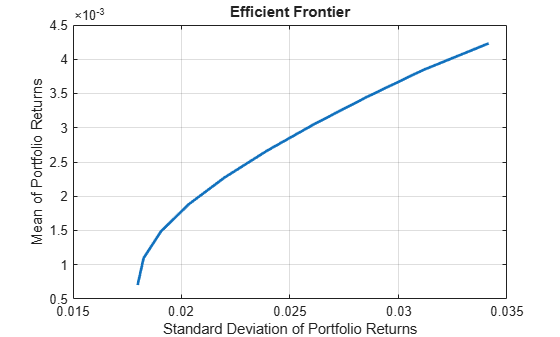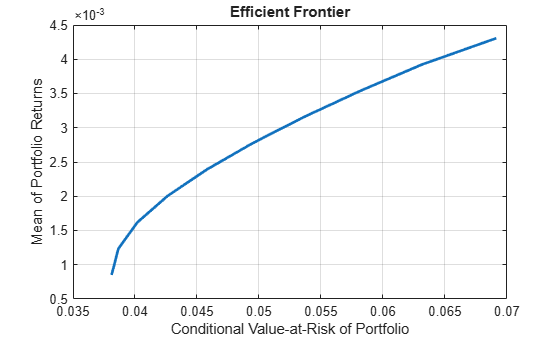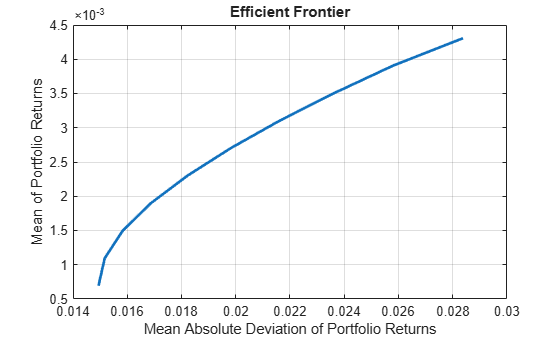# estimateFrontier

Estimate specified number of optimal portfolios on the efficient frontier

## Syntax

``````[pwgt,pbuy,psell] = estimateFrontier(obj)``````
``````[pwgt,pbuy,psell] = estimateFrontier(obj,NumPorts)``````

## Description

example

``````[pwgt,pbuy,psell] = estimateFrontier(obj)``` estimates the specified number of optimal portfolios on the efficient frontier for `Portfolio`, `PortfolioCVaR`, or `PortfolioMAD` objects. For details on the respective workflows when using these different objects, see Portfolio Object Workflow, PortfolioCVaR Object Workflow, and PortfolioMAD Object Workflow.```

example

``````[pwgt,pbuy,psell] = estimateFrontier(obj,NumPorts)``` estimates the specified number of optimal portfolios on the efficient frontier with an additional option specified for `NumPorts`. ```

## Examples

collapse all

Create efficient portfolios:

```load CAPMuniverse p = Portfolio('AssetList',Assets(1:12)); p = estimateAssetMoments(p, Data(:,1:12),'missingdata',true); p = setDefaultConstraints(p); plotFrontier(p);``````pwgt = estimateFrontier(p, 5); pnames = cell(1,5); for i = 1:5 pnames{i} = sprintf('Port%d',i); end Blotter = dataset([{pwgt},pnames],'obsnames',p.AssetList); disp(Blotter);```
``` Port1 Port2 Port3 Port4 Port5 AAPL 0.017926 0.058247 0.097816 0.12955 0 AMZN 0 0 0 0 0 CSCO 0 0 0 0 0 DELL 0.0041906 0 0 0 0 EBAY 0 0 0 0 0 GOOG 0.16144 0.35678 0.55228 0.75116 1 HPQ 0.052566 0.032302 0.011186 0 0 IBM 0.46422 0.36045 0.25577 0.11928 0 INTC 0 0 0 0 0 MSFT 0.29966 0.19222 0.082949 0 0 ORCL 0 0 0 0 0 YHOO 0 0 0 0 0 ```

Create a `Portfolio` object for 12 stocks based on `CAPMuniverse.mat`.` `

```load CAPMuniverse p0 = Portfolio('AssetList',Assets(1:12)); p0 = estimateAssetMoments(p0, Data(:,1:12),'missingdata',true); p0 = setDefaultConstraints(p0);```

Use `setMinMaxNumAssets` to define a maximum number of 3 assets.

`p1 = setMinMaxNumAssets(p0, [], 3);`

Use `setBounds` to define a lower and upper bound and a `BoundType` of `'Conditional'`.

```p1 = setBounds(p1, 0.1, 0.5,'BoundType', 'Conditional'); pwgt = estimateFrontier(p1, 5); ```

The following table shows that the optimized allocations only have maximum 3 assets invested, and small positions less than 0.1 are avoided.

`result = table(p0.AssetList', pwgt)`
```result=12×2 table Var1 pwgt ________ ___________________________________________________ {'AAPL'} 0 0 0 0.14301 0 {'AMZN'} 0 0 0 0 0 {'CSCO'} 0 0 0 0 0 {'DELL'} 0 0 0 0 0 {'EBAY'} 0 0 0 0 0.5 {'GOOG'} 0.16981 0.29588 0.42214 0.49999 0.5 {'HPQ' } 0 0 0 0 0 {'IBM' } 0.49592 0.43629 0.37308 0.35699 0 {'INTC'} 0 0 0 0 0 {'MSFT'} 0.33426 0.26783 0.20478 0 0 {'ORCL'} 0 0 0 0 0 {'YHOO'} 0 0 0 0 0 ```

The `estimateFrontier` function uses the MINLP solver to solve this problem. Use the `setSolverMINLP` function to configure the `SolverType` and options.

`p1.solverTypeMINLP`
```ans = 'OuterApproximation' ```
`p1.solverOptionsMINLP`
```ans = struct with fields: MaxIterations: 1000 AbsoluteGapTolerance: 1.0000e-07 RelativeGapTolerance: 1.0000e-05 NonlinearScalingFactor: 1000 ObjectiveScalingFactor: 1000 Display: 'off' CutGeneration: 'basic' MaxIterationsInactiveCut: 30 ActiveCutTolerance: 1.0000e-07 IntMasterSolverOptions: [1x1 optim.options.Intlinprog] NumIterationsEarlyIntegerConvergence: 30 ```

Create efficient portfolios:

```load CAPMuniverse p = PortfolioCVaR('AssetList',Assets(1:12)); p = simulateNormalScenariosByData(p, Data(:,1:12), 20000 ,'missingdata',true); p = setDefaultConstraints(p); p = setProbabilityLevel(p, 0.95); plotFrontier(p);``````pwgt = estimateFrontier(p, 5); pnames = cell(1,5); for i = 1:5 pnames{i} = sprintf('Port%d',i); end Blotter = dataset([{pwgt},pnames],'obsnames',p.AssetList); disp(Blotter);```
``` Port1 Port2 Port3 Port4 Port5 AAPL 0.010562 0.07364 0.11931 0.13073 0 AMZN 0 0 0 0 0 CSCO 0 0 0 0 0 DELL 0.022649 0 0 0 0 EBAY 0 0 0 0 0 GOOG 0.203 0.38011 0.56202 0.75919 1 HPQ 0.042772 0.0094711 0 0 0 IBM 0.44444 0.36456 0.26305 0.11009 0 INTC 0 0 0 0 0 MSFT 0.27658 0.17222 0.055624 0 0 ORCL 0 0 0 0 0 YHOO 0 0 0 0 0 ```

Create efficient portfolios:

```load CAPMuniverse p = PortfolioMAD('AssetList',Assets(1:12)); p = simulateNormalScenariosByData(p, Data(:,1:12), 20000 ,'missingdata',true); p = setDefaultConstraints(p); plotFrontier(p);``````pwgt = estimateFrontier(p, 5); pnames = cell(1,5); for i = 1:5 pnames{i} = sprintf('Port%d',i); end Blotter = dataset([{pwgt},pnames],'obsnames',p.AssetList); disp(Blotter);```
``` Port1 Port2 Port3 Port4 Port5 AAPL 0.029787 0.076199 0.11265 0.13397 0 AMZN 0 0 0 0 0 CSCO 0 0 0 0 0 DELL 0.0089177 0 0 0 0 EBAY 0 0 0 0 0 GOOG 0.16094 0.3516 0.54479 0.74898 1 HPQ 0.056856 0.023073 0 0 0 IBM 0.46074 0.37919 0.29379 0.11705 0 INTC 0 0 0 0 0 MSFT 0.28277 0.16994 0.048762 0 0 ORCL 0 0 0 0 0 YHOO 0 0 0 0 0 ```

Obtain the default number of efficient portfolios over the entire range of the efficient frontier.

```m = [ 0.05; 0.1; 0.12; 0.18 ]; C = [ 0.0064 0.00408 0.00192 0; 0.00408 0.0289 0.0204 0.0119; 0.00192 0.0204 0.0576 0.0336; 0 0.0119 0.0336 0.1225 ]; p = Portfolio; p = setAssetMoments(p, m, C); p = setDefaultConstraints(p); pwgt = estimateFrontier(p); disp(pwgt);```
``` Columns 1 through 7 0.8891 0.7215 0.5540 0.3865 0.2190 0.0515 0 0.0369 0.1289 0.2209 0.3129 0.4049 0.4969 0.4049 0.0404 0.0567 0.0730 0.0893 0.1056 0.1219 0.1320 0.0336 0.0929 0.1521 0.2113 0.2705 0.3297 0.4630 Columns 8 through 10 0 0 0 0.2314 0.0579 0 0.1394 0.1468 0 0.6292 0.7953 1.0000 ```

Starting from the initial portfolio, the `estimateFrontier` function returns purchases and sales to get from your initial portfolio to each efficient portfolio on the efficient frontier. Given an initial portfolio in `pwgt0`, you can obtain purchases and sales.

```m = [ 0.05; 0.1; 0.12; 0.18 ]; C = [ 0.0064 0.00408 0.00192 0; 0.00408 0.0289 0.0204 0.0119; 0.00192 0.0204 0.0576 0.0336; 0 0.0119 0.0336 0.1225 ]; p = Portfolio; p = setAssetMoments(p, m, C); p = setDefaultConstraints(p); pwgt0 = [ 0.3; 0.3; 0.2; 0.1 ]; p = setInitPort(p, pwgt0); [pwgt, pbuy, psell] = estimateFrontier(p); display(pwgt);```
```pwgt = 4×10 0.8891 0.7215 0.5540 0.3865 0.2190 0.0515 0 0 0 0 0.0369 0.1289 0.2209 0.3129 0.4049 0.4969 0.4049 0.2314 0.0579 0 0.0404 0.0567 0.0730 0.0893 0.1056 0.1219 0.1320 0.1394 0.1468 0 0.0336 0.0929 0.1521 0.2113 0.2705 0.3297 0.4630 0.6292 0.7953 1.0000 ```
`display(pbuy);`
```pbuy = 4×10 0.5891 0.4215 0.2540 0.0865 0 0 0 0 0 0 0 0 0 0.0129 0.1049 0.1969 0.1049 0 0 0 0 0 0 0 0 0 0 0 0 0 0 0 0.0521 0.1113 0.1705 0.2297 0.3630 0.5292 0.6953 0.9000 ```
`display(psell);`
```psell = 4×10 0 0 0 0 0.0810 0.2485 0.3000 0.3000 0.3000 0.3000 0.2631 0.1711 0.0791 0 0 0 0 0.0686 0.2421 0.3000 0.1596 0.1433 0.1270 0.1107 0.0944 0.0781 0.0680 0.0606 0.0532 0.2000 0.0664 0.0071 0 0 0 0 0 0 0 0 ```

Obtain the default number of efficient portfolios over the entire range of the efficient frontier.

```m = [ 0.05; 0.1; 0.12; 0.18 ]; C = [ 0.0064 0.00408 0.00192 0; 0.00408 0.0289 0.0204 0.0119; 0.00192 0.0204 0.0576 0.0336; 0 0.0119 0.0336 0.1225 ]; m = m/12; C = C/12; rng(11); AssetScenarios = mvnrnd(m, C, 20000); p = PortfolioCVaR; p = setScenarios(p, AssetScenarios); p = setDefaultConstraints(p); p = setProbabilityLevel(p, 0.95); pwgt = estimateFrontier(p); disp(pwgt);```
``` Columns 1 through 7 0.8451 0.6849 0.5159 0.3541 0.1903 0.0323 0 0.0613 0.1429 0.2291 0.3165 0.3983 0.4721 0.3528 0.0451 0.0634 0.0944 0.1082 0.1340 0.1580 0.1736 0.0485 0.1089 0.1606 0.2213 0.2775 0.3376 0.4736 Columns 8 through 10 0 0 0 0.1804 0 0 0.1918 0.2212 0 0.6277 0.7788 1.0000 ```

The function `rng`($seed$) resets the random number generator to produce the documented results. It is not necessary to reset the random number generator to simulate scenarios.

Starting from the initial portfolio, the `estimateFrontier` function returns purchases and sales to get from your initial portfolio to each efficient portfolio on the efficient frontier. Given an initial portfolio in `pwgt0`, you can obtain purchases and sales.

```m = [ 0.05; 0.1; 0.12; 0.18 ]; C = [ 0.0064 0.00408 0.00192 0; 0.00408 0.0289 0.0204 0.0119; 0.00192 0.0204 0.0576 0.0336; 0 0.0119 0.0336 0.1225 ]; m = m/12; C = C/12; rng(11); AssetScenarios = mvnrnd(m, C, 20000); p = PortfolioCVaR; p = setScenarios(p, AssetScenarios); p = setDefaultConstraints(p); p = setProbabilityLevel(p, 0.95); pwgt0 = [ 0.3; 0.3; 0.2; 0.1 ]; p = setInitPort(p, pwgt0); [pwgt, pbuy, psell] = estimateFrontier(p); display(pwgt);```
```pwgt = 4×10 0.8451 0.6849 0.5159 0.3541 0.1903 0.0323 0 0 0 0 0.0613 0.1429 0.2291 0.3165 0.3983 0.4721 0.3528 0.1804 0 0 0.0451 0.0634 0.0944 0.1082 0.1340 0.1580 0.1736 0.1918 0.2212 0 0.0485 0.1089 0.1606 0.2213 0.2775 0.3376 0.4736 0.6277 0.7788 1.0000 ```
`display(pbuy);`
```pbuy = 4×10 0.5451 0.3849 0.2159 0.0541 0 0 0 0 0 0 0 0 0 0.0165 0.0983 0.1721 0.0528 0 0 0 0 0 0 0 0 0 0 0 0.0212 0 0 0.0089 0.0606 0.1213 0.1775 0.2376 0.3736 0.5277 0.6788 0.9000 ```
`display(psell);`
```psell = 4×10 0 0 0 0 0.1097 0.2677 0.3000 0.3000 0.3000 0.3000 0.2387 0.1571 0.0709 0 0 0 0 0.1196 0.3000 0.3000 0.1549 0.1366 0.1056 0.0918 0.0660 0.0420 0.0264 0.0082 0 0.2000 0.0515 0 0 0 0 0 0 0 0 0 ```

The function `rng`($seed$) resets the random number generator to produce the documented results. It is not necessary to reset the random number generator to simulate scenarios.

Obtain the default number of efficient portfolios over the entire range of the efficient frontier.

```m = [ 0.05; 0.1; 0.12; 0.18 ]; C = [ 0.0064 0.00408 0.00192 0; 0.00408 0.0289 0.0204 0.0119; 0.00192 0.0204 0.0576 0.0336; 0 0.0119 0.0336 0.1225 ]; m = m/12; C = C/12; rng(11); AssetScenarios = mvnrnd(m, C, 20000); p = PortfolioMAD; p = setScenarios(p, AssetScenarios); p = setDefaultConstraints(p); pwgt = estimateFrontier(p); disp(pwgt);```
``` Columns 1 through 7 0.8817 0.7150 0.5488 0.3812 0.2167 0.0494 0 0.0431 0.1285 0.2127 0.2993 0.3832 0.4679 0.3599 0.0387 0.0604 0.0826 0.1047 0.1237 0.1480 0.1799 0.0366 0.0961 0.1559 0.2148 0.2763 0.3348 0.4601 Columns 8 through 10 0 0 0 0.1758 0 0 0.2091 0.2266 0 0.6151 0.7734 1.0000 ```

The function `rng`($seed$) resets the random number generator to produce the documented results. It is not necessary to reset the random number generator to simulate scenarios.

Starting from the initial portfolio, the `estimateFrontier` function returns purchases and sales to get from your initial portfolio to each efficient portfolio on the efficient frontier. Given an initial portfolio in `pwgt0`, you can obtain purchases and sales.

```m = [ 0.05; 0.1; 0.12; 0.18 ]; C = [ 0.0064 0.00408 0.00192 0; 0.00408 0.0289 0.0204 0.0119; 0.00192 0.0204 0.0576 0.0336; 0 0.0119 0.0336 0.1225 ]; m = m/12; C = C/12; rng(11); AssetScenarios = mvnrnd(m, C, 20000); p = PortfolioMAD; p = setScenarios(p, AssetScenarios); p = setDefaultConstraints(p); pwgt0 = [ 0.3; 0.3; 0.2; 0.1 ]; p = setInitPort(p, pwgt0); [pwgt, pbuy, psell] = estimateFrontier(p); display(pwgt);```
```pwgt = 4×10 0.8817 0.7150 0.5488 0.3812 0.2167 0.0494 0 0 0 0 0.0431 0.1285 0.2127 0.2993 0.3832 0.4679 0.3599 0.1758 0 0 0.0387 0.0604 0.0826 0.1047 0.1237 0.1480 0.1799 0.2091 0.2266 0 0.0366 0.0961 0.1559 0.2148 0.2763 0.3348 0.4601 0.6151 0.7734 1.0000 ```
`display(pbuy);`
```pbuy = 4×10 0.5817 0.4150 0.2488 0.0812 0 0 0 0 0 0 0 0 0 0 0.0832 0.1679 0.0599 0 0 0 0 0 0 0 0 0 0 0.0091 0.0266 0 0 0 0.0559 0.1148 0.1763 0.2348 0.3601 0.5151 0.6734 0.9000 ```
`display(psell);`
```psell = 4×10 0 0 0 0 0.0833 0.2506 0.3000 0.3000 0.3000 0.3000 0.2569 0.1715 0.0873 0.0007 0 0 0 0.1242 0.3000 0.3000 0.1613 0.1396 0.1174 0.0953 0.0763 0.0520 0.0201 0 0 0.2000 0.0634 0.0039 0 0 0 0 0 0 0 0 ```

The function `rng`($seed$) resets the random number generator to produce the documented results. It is not necessary to reset the random number generator to simulate scenarios.

## Input Arguments

collapse all

Object for portfolio, specified using `Portfolio`, `PortfolioCVaR`, or `PortfolioMAD` object. For more information on creating a portfolio object, see

Data Types: `object`

Number of points to obtain on the efficient frontier, specified as a scalar integer.

### Note

If no value is specified for `NumPorts`, the default value is obtained from the hidden property `defaultNumPorts` (default value is `10`). If `NumPorts` = `1`, this function returns the portfolio specified by the hidden property `defaultFrontierLimit` (current default value is `'min'`).

Data Types: `double`

## Output Arguments

collapse all

Optimal portfolios on the efficient frontier with specified number of portfolios spaced equally from minimum to maximum portfolio return, returned as a `NumAssets`-by-`NumPorts` matrix. `pwgt` is returned for a `Portfolio`, `PortfolioCVaR`, or `PortfolioMAD` input object (`obj`).

Purchases relative to an initial portfolio for optimal portfolios on the efficient frontier, returned as `NumAssets`-by-`NumPorts` matrix.

### Note

If no initial portfolio is specified in `obj.InitPort`, that value is assumed to be `0` such that `pbuy = max(0, pwgt)` and `psell = max(0, -pwgt)`.

`pbuy` is returned for a `Portfolio`, `PortfolioCVaR`, or `PortfolioMAD` input object (`obj`).

Sales relative to an initial portfolio for optimal portfolios on the efficient frontier, returned as a `NumAssets`-by-`NumPorts` matrix.

### Note

If no initial portfolio is specified in `obj.InitPort`, that value is assumed to be `0` such that `pbuy = max(0, pwgt)` and `psell = max(0, -pwgt)`.

`psell` is returned for `Portfolio`, `PortfolioCVaR`, or `PortfolioMAD` input object (`obj`).

## Tips

• You can also use dot notation to estimate the specified number of optimal portfolios over the entire efficient frontier.

` [pwgt, pbuy, psell] = obj.estimateFrontier(NumPorts);`

• When introducing transaction costs and turnover constraints to the `Portfolio`, `PortfolioCVaR`, or `PortfolioMAD` object, the portfolio optimization objective contains a term with an absolute value. For more information on how Financial Toolbox™ handles such cases algorithmically, see References.

 Cornuejols, G., and R. Tutuncu. Optimization Methods in Finance. Cambridge University Press, 2007.